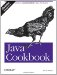# Recipe 5.13 Generating Random Numbers

#### Problem

You need to generate random numbers in a hurry.

#### Solution

Use java.lang.Math.random( ) to generate random numbers. There is no claim that the random values it returns are very good random numbers, however. This code exercises the random( ) method:

`// Random1.java  // java.lang.Math.random( ) is static, don't need to construct Math  System.out.println("A random from java.lang.Math is " + Math.random( ));`

Note that this method only generates double values. If you need integers, you need to scale and round:

`/** Generate random ints by asking Random( ) for  * a series of random integers from 1 to 10, inclusive.  *  * @author Ian Darwin, http://www.darwinsys.com/  * @version \$Id: ch05.xml,v 1.5 2004/05/04 20:1:35 ian Exp \$  */ public class RandomInt {     public static void main(String[] a) {         Random r = new Random( );         for (int i=0; i<1000; i++)             // nextInt(10) goes from 0-9; add 1 for 1-10;             System.out.println(1+Math.round(r.nextInt(10)));     } }`

To see if it was really working well, I used the Unix tools sort and uniq, which, together, give a count of how many times each value was chosen. For 1,000 integers, each of 10 values should be chosen about 100 times:

`C:> java RandomInt | sort -n | uniq -c  110 1  106 2   98 3  109 4  108 5   99 6   94 7   91 8   94 9   91 10 C:>`

Recipe 5.14 shows easier and better ways to get random integers and doubles. Also see the Javadoc documentation for java.lang.Math and the warning in this chapter's Introduction about pseudo-randomness versus real randomness.Java Cookbook, Second Edition
ISBN: 0596007019
EAN: 2147483647
Year: 2003
Pages: 409
Authors: Ian F Darwin

Similar book on Amazon